close

# The Answer to Your PowerShell Question: Working with ObjectsToday’s article is yet another guide to get you thinking about working with objects in PowerShell. To illustrate, here’s a question I came across in a PowerShell forum.

How would I write a PowerShell script, which takes an amount, adds 15% for example, and displays the amount, the 15% addition, and the total?

I really don’t have any idea of the need for such a tool, but that’s okay. Instead, let’s use this as a learning opportunity.
In reading this question, I get the sense the user is looking for ways to present text values. Back in the day we would have used a VBScript file like this:

```dim Amount,Interest,Increase,Total
Amount = 100
Interest = 15
Increase = (Interest/100)*Amount
Total = Amount+Increase
wscript.echo "Amount = " & Amount
wscript.echo "Interest = " & Interest
wscript.echo "Increase = " & Increase
wscript.echo "Total = " & Total
```

Running this would give us a simple result: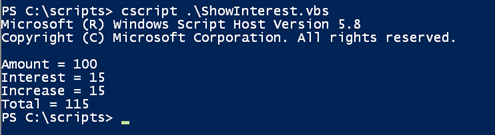A VBScript solution (Image Credit: Jeff Hicks)

Clearly that is not what we want in PowerShell, although it would be easy to powershell-ize the VBScript, which I’ve seen some people do. Let’s start with an equivalent set of PowerShell variables.

```\$amount = 100
\$interest= .15
```

Notice that in this example the variable interest is expressed as a decimal equivalent. I can use Select-Object with custom hashtables to write an object to the pipeline with all of the relevant information.

```\$amount | select @{Name="Value";Expression={\$_}},
@{Name="Interest";Expression={\$interest*100}},
@{Name="Increase";Expression = {\$_ * \$interest}},
@{Name="Total";Expression={ \$_ + (\$_ * \$interest) }}
```

The Expression scriptblock for each entry performs some type of calculation.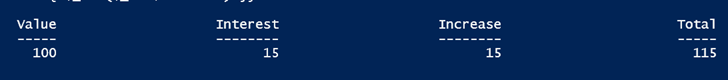An object result (Image Credit: Jeff Hicks)

I suppose I should point out that because \$Amount is a number, it doesn’t have any properties. So I created one called value, which uses the object itself (\$_).

While Select-Object technically is creating a custom object behind the scenes, you can create the object yourself, which might be a little easier to follow. Again, I start with some initial values:

```\$amount = 100
\$interest = .15
```

For the sake of clarity, I’ll create additional variables for the increase and new total.

```\$increase = \$amount * \$interest
\$newAmount = \$amount + \$increase
```

Finally, I can create a new object. There are several techniques, but I’ll use the [pscustomobject] to keep it simple.

```[pscustomObject]@{
Amount = \$amount
Percent = \$interest * 100
Increase = \$Increase
Total = \$newAmount
}
```

The result is the same: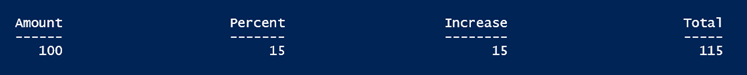PSCustomObject result (Image Credit: Jeff Hicks)

Now that I know to present the data the way I want it, I can build a simple function to make it re-usable.

```Function Invoke-Increase {
Param([double]\$Amount,[double]\$Percent)
#convert the percent into the correct value
\$increase = \$amount * (\$percent/100)
#create an object
[pscustomObject]@{
Amount = \$amount
Percent = \$percent
Increase = \$Increase
Total = \$amount + \$increase
}
} #end function
```

I added parameters so I can specify the amount and percent increase, what I had been referring to as “interest.” I cast the variables as the type [double], so that I could pass values like 123.98.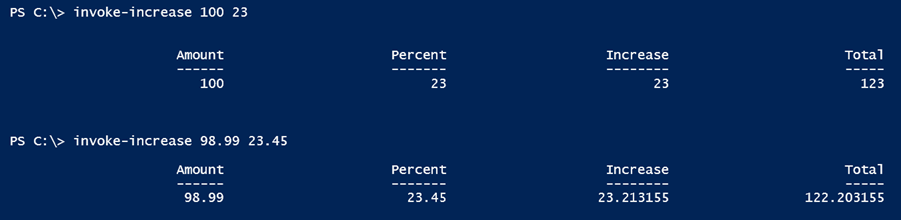Testing the function (Image Credit: Jeff Hicks)

I now have a re-usable tool that writes an object to the pipeline that contains the necessary information.
One thing that always comes up when I show things like this is someone will ask about formatting the decimal places. OK. Add that to your function. Here’s a revised version that uses the Round() method from the [Math] class that rounds the specified number of decimal places. I set a default value of 2.

```Function Invoke-Increase {
Param([double]\$Amount,[double]\$Percent,[int]\$Decimal = 2)
#convert the percent into the correct value
\$increase = \$amount * (\$percent/100)
#create an object
[pscustomObject]@{
Amount = \$amount
Percent = \$percent
Increase = [math]::Round(\$Increase,\$Decimal)
Total = [math]::Round(\$amount + \$increase,\$Decimal)
}
} #end function
```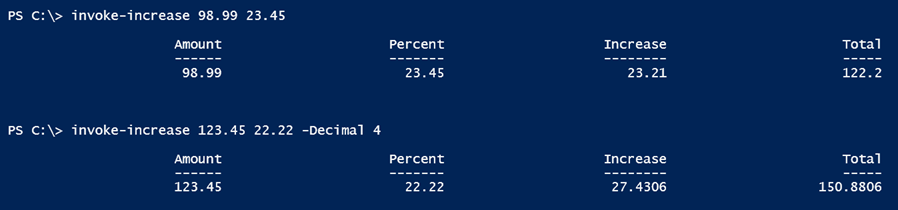Using the revised function (Image Credit: Jeff Hicks)

This should be enough to get you started and realizing that PowerShell is the answer, especially when you stop thinking about text output. Of course, there are some alternative techniques you can use but I think I’ve run out of time for today so check back later for the wrap-up to this mini-series.

More in PowerShell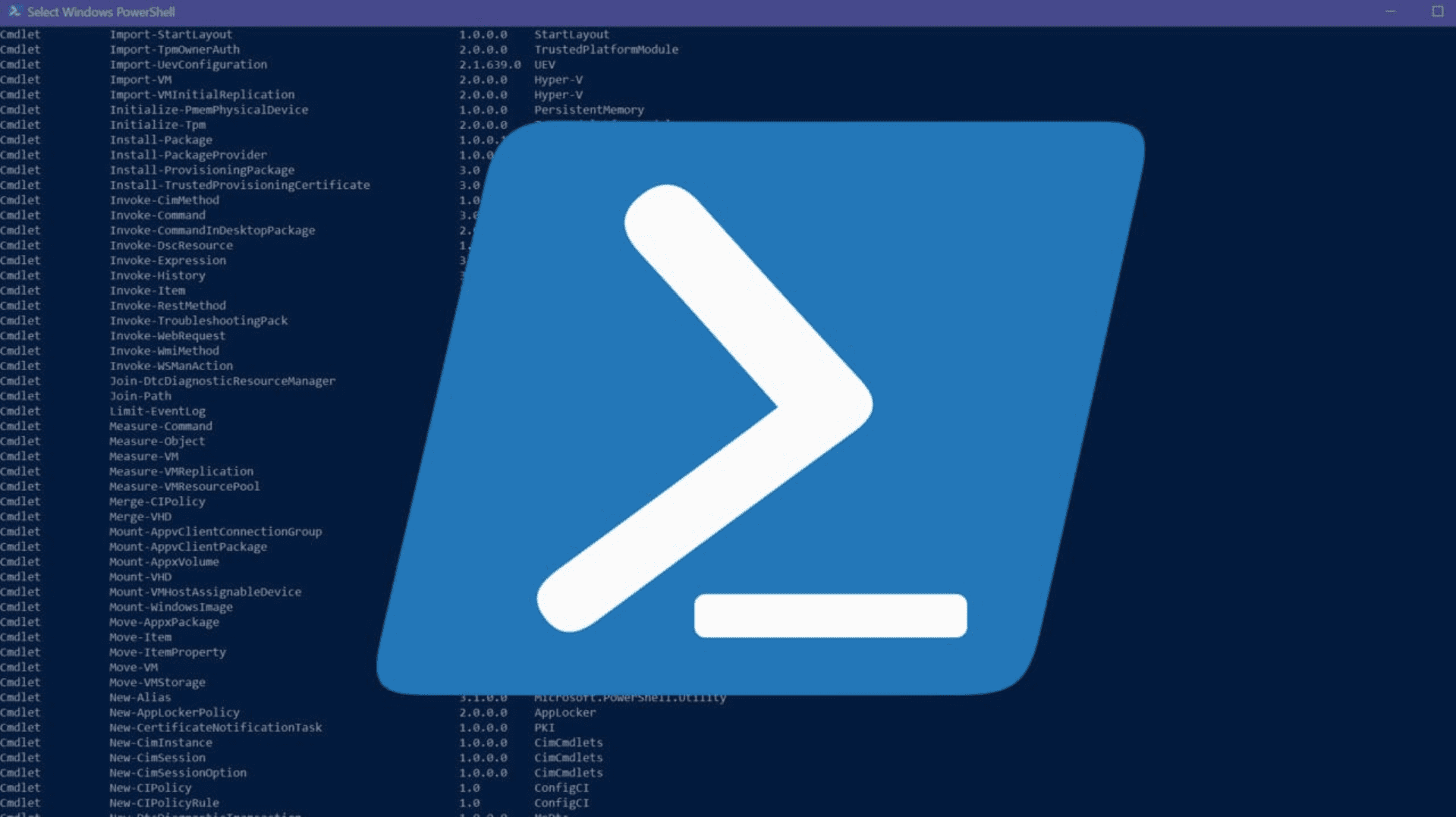What is PowerShell and How to Get Started With It?

Sep 7, 2022 | Mike KanakosUse a PowerShell Substring to Search Inside a String

Aug 11, 2022 | Jeff HicksTest Network Connectivity with PowerShell Test-Connection

Aug 9, 2022 | Jeff HicksHow to Use the PowerShell Exit Keyword to Terminate Scripts

Aug 8, 2022 | Ivan MirchevError Handling With PowerShell Try Catch Blocks

Aug 5, 2022 | Adam BertramHow to Use PowerShell Grep: Select-String and RegEx Real World Examples

Aug 2, 2022 | Jeff Hicks

Most popular on petri

Article saved!

Access saved content from your profile page. View Saved